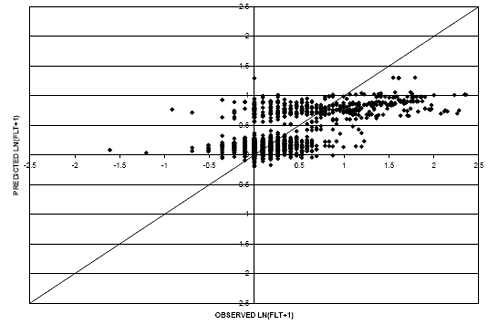U.S. Department of Transportation
1200 New Jersey Avenue, SE
Washington, DC 20590
202-366-4000

Federal Highway Administration Research and Technology
Coordinating, Developing, and Delivering Highway Transportation InnovationsThis report is an archived publication and may contain dated technical, contact, and link information
 Federal Highway Administration > Publications > Research Publications > LTPP Publications > 06121 > Chapt5.Cfm > Long-Term Pavement Performance (LTPP) Data Analysis Support: National Pooled Fund Study Tpf-5(013)
 Publication Number: FHWA-HRT-06-121 Date: November 2006

# Long-Term Pavement Performance (LTPP) Data Analysis Support: National Pooled Fund Study Tpf-5(013)

## Chapter 5. Performance Model Development and Selection

As described in the previous section, simple statistical tools such as maximum, minimum,average, and standard deviation were reviewed to identify data acquisition errors or problems with the calculations performed. Box plots, frequency graphs, and residual plots were also created to develop an understanding of the datasets and to study interaction,correlation, and the type of distribution present in the data. These tools were also used to verify whether the nature of the data violate any statistical assumptions made during the analysis. As part of this initial statistical review, the dataset was also inspected to identify and remove data collected after unrecorded pavement improvements were performed.Criteria were developed for each performance measure and applied to the dataset to flag instances of significant reductions in deterioration. Table 18 summarizes the checks used in this process.

Table 18. Criteria to warrant additional investigation of unrecorded pavement improvements.
Pavement TypePerformance MeasureReduction Criteria that Warrant Investigation
AC IRI>0.4 m/km (25.4 inches/mi) reduction
AC DISTRESS>30% reduction in sum of key distress typesa
AC RUTDEPTH>10 mm (0.4 inch) reduction
PCC IRI>0.4 m/km (25.4 inches/mi) reduction
PCC DISTRESS>30% reduction in sum of key distress typesb
PCC FAULTING>2 mm (0.08 inch) reduction
 aDeduct values of fatigue cracking, block cracking, longitudinal wheelpath cracking,longitudinal nonwheelpath cracking, transverse cracking, and patching were summed for this evaluation. bNormalized quantities of corner breaks, longitudinal cracking, transverse cracking, and patching were summed for this evaluation.

Each test section flagged was thoroughly examined using all performance measures to determine if the reduction was most likely caused by an improvement to the pavement or if it could be attributed to data variability. In general, if the majority of the performance measures demonstrated a reduction in deterioration, it was concluded that an unreported pavement improvement was applied. Data collected after the improvement were removed from the dataset.

Due to the subjective nature of distress data, additional quality reviews were performed on the data to remove records where a reduction was observed in one of the distress types as a result of rater variability. For example, distress data collected at test section 181037 at pavement age equal to 10.2 years recorded a BC deduct value of 74.3 (and 0 values for TC and FWPC). The next distress survey (11.4 years) recorded a decreased BC value of 33.2; however, the values of TC and FWPC increased significantly (values of 68 and 7.5, respectively). The first surveyor rated the distress as BC while the second rater opted to rate a series of longitudinal and transverse cracks. As such, the BC data recorded at 11.4 years were removed from the dataset.

Test sections with known construction issues were also removed from the dataset. One such example is the Nevada SPS-2 project that experienced excessive cracking just after construction. These types of issues could not be accounted for in the models, and they would simply add variability to the analysis.

Upon completion of the review, work began on developing regression models. Two regression methods were considered for use in the study: the general linear model (GLM) and the robust regression model. The GLM is susceptible to extreme outlying cases that cannot be definitively determined as erroneous data. Because this project incorporates national data with many contributing factors, extreme cases do exist that cannot be established as errors, and they need to be accounted for in the model. The robust regression techniques dampen the effect of these extreme cases by applying a weighting factor based on residuals. The robust model is used to make adjustments to the GLM and validate the model using an iterative process.

Figure 7 is a plot of student residuals as a function of Hat values,(15) which is a graphical tool to evaluate observations in a dataset. The student residual is the ratio of a residual to its standard error. Large absolute values of the student residual (larger than 2.5) are an indication of outliers in the data. The Hat diagonal refers to the diagonal elements of the Hat matrix in the least squares estimation,(16) and it quantifies the leverage of each observation on the predicted value for that observation. The cluster of points located further to the right in figure 7 is a group of influential observations.

Figure 7. Scatter plot. Outlier-influential observation detection plot.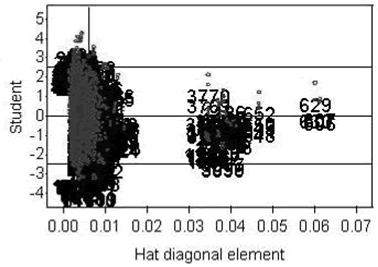The robust method should be used if these influential observations are truly outliers or questionable data points that would negatively affect the model. On the other hand, these cases could be treated as any other with their full effect incorporated into the model (the GLM method) if they are valid data points that represent extreme conditions.

To determine which method is more appropriate and will produce the most representative model, the nature of the dataset, as well as the number of quality control checks performed on the data, must be considered. If extreme cases are expected (given the design of the experiment) and a rigorous quality review has been performed on the data, it is highly probable that the remaining influential observations are valid, and reducing their impact on the model would bias the model’s prediction capability.

For this study, data come from a national database in which some of the variables may be set to extreme limits resulting in extreme performance observations. On a small scale, the SPS-1 projects can be used to illustrate this. Each of the 12 test sections at an SPS-1 project has a different structural capacity, but all experience the same traffic loading. By experimental design, certain variables (in the case of SPS-1 projects, the ratio of traffic loading to structural capacity) would be set to the extreme ends of the spectrum. As such, extreme observations are to be expected in the dataset, and they are necessary to generate a model that reflects observed performance.

In addition, the analysis team performed considerable logical quality review on the data to identify and remove data that were believed to be erroneous. The data have also undergone the quality control process used by the LTPP team before releasing the data for public use. It is unlikely that the remaining influential observations are erroneous or unrepresentative of the dataset.

To further compare the two methodologies, two models were developed for absolute IRI of asphalt pavements. One model was developed using the robust method while the other used the GLM procedure. The predicted IRI values from the robust and GLM models versus the observed IRI values can be found in figures 8 and 9, respectively. The GLM method produces a model that has less bias than the robust model. In figure 8, the majority of the data points are clustered below the line of equality (circled in the figure). The cluster of the GLM model is more centered on the equality line compared with the robust model. This indicates that the robust method of reducing the effect of extreme observations results in a model that generally predicts values less than the observed values.

Considering the nature of the dataset, the level of quality reviews performed on the data, and the results from the previous comparison, the GLM method was chosen to develop regression models for this study.

Figure 8. Scatter plot. Observed versus predicted values of absolute IRI (shifted) using the robust method.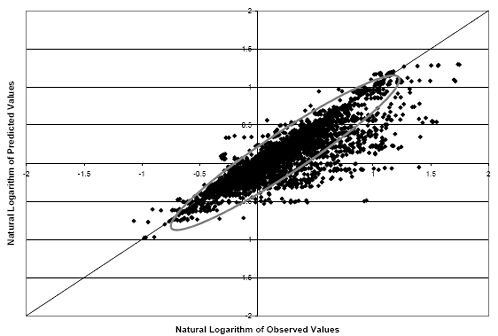Figure 9. Scatter plot. Observed versus predicted values of absolute IRI (shifted) using the GLM method.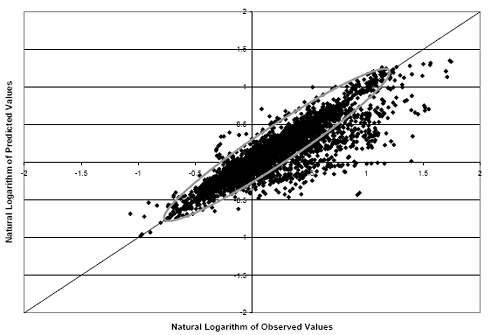### PAVEMENT ROUGHNESS PREDICTION MODELS

It is well known that postconstruction roughness varies from one project to the next because of differences such as construction techniques and specifications. These differences also significantly affect the progression of roughness over time, and could add variability to the model. To counter this, the analysis team investigated models to predict change in IRI as the performance measure. As described previously, change in IRI was calculated by subtracting the first LTPP measurement from each of the subsequent measurements. Through this process, it was believed that the postconstruction differences were inherent to the initial IRI measurement and would be removed from the subsequent measurements.

The resultant models, however, did not provide a good correlation with the observed dataset. The lack of fit can be partially contributed to the differences in age at which the first LTPP measurement was taken. For example, the first IRI measurement at test section 086002 was taken at an age of 21.3 years while the initial measurement at test section 100101 was taken at 1.1 years. The reference measurement used to calculate change in IRI for subsequent measurements was captured at different ages as well as at locations on the deterioration curve which was not accounted for in the model.

Based on the observations made using the change in IRI, a decision was made to develop regression models using absolute IRI. To account for the postconstruction differences in roughness, initial IRI and the age of initial IRI measurement were incorporated as explanatory variables in the model. The models for this performance measure provided a better correlation than the change in IRI measure. Figure 10 provides a graph of actual values measured at test section 307066 along with values predicted by the model. As can be seen in the graph, the model is predicting the accumulation of roughness with time fairly accurately (indicated by equivalent slopes), but the model is offset from the actual measurements. Although only one example is shown, this offset was observed in many cases and varied for each test section. These differences can be reduced or eliminated by shifting the model to predict the initial IRI at the corresponding age of initial IRI measurement. The shifted model for test section 307066 can be found in figure 11.

To further evaluate the prediction capability of the model, two scatter plots were generated using the flexible dataset. A scatter plot for the regression model (without shifting) is shown in figure 12, while the scatter plot for the shifted regression model is shown in figure 13. As can be seen from the figures, shifting the predicted values based on the initial IRI value results in an improvement in the model’s accuracy.

Furthermore, the root mean squared error (RMSE) for the regression model (without shifting) was 0.18, while the shifted model exhibited a RMSE value of 0.17. RMSE is used to make relative comparisons on the “goodness of fit” between two models predicting the same performance measure (from the same dataset). Lower RMSE values are indicative of a model that represents the observed values better.

In consideration of the reasons discussed, it was determined that the shifted model provided a better representation of the observed values in the dataset, and therefore, the shifting methodology was used to predict pavement roughness over time for flexible pavements.

Figure 10. Graph. Example of predicted (without shifting) and observed values for test section 307066.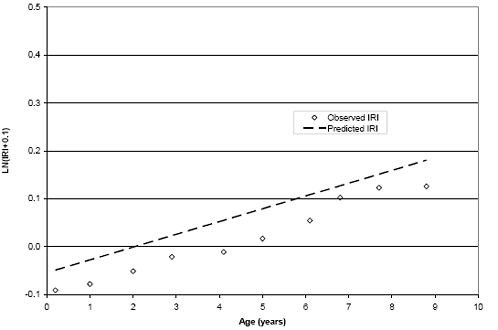Figure 11. Graph. Example of predicted (shifted) and observed values for test section 307066.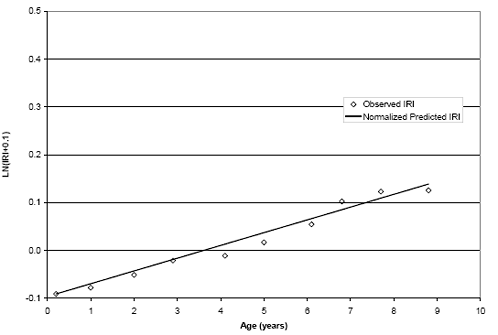Figure 12. Scatter plot. Flexible IRI model without shifting.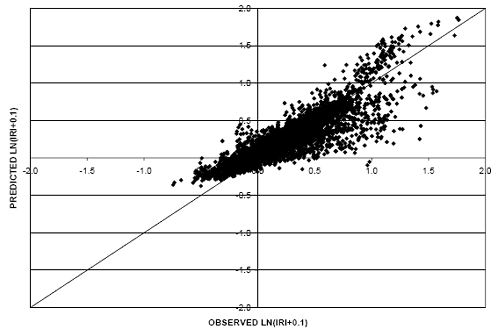Figure 13. Scatter plot. Flexible IRI model (shifted).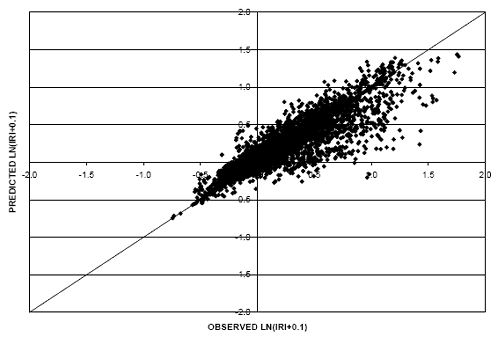The same exercise was performed for rigid pavement roughness to determine if shifting the model made an improvement on its prediction capability. Figures 14 and 15 provide scatter plots for the model (without shifting) and the shifted model, respectively. In this case, the model (without shifting) resulted in a lower RMSE value (0.14) than the shifted model (RMSE equal to 0.15). Therefore, comparisons on rigid pavements were made using the model that was not shifted.

Figure 14. Scatter plot. Rigid IRI model without shifting.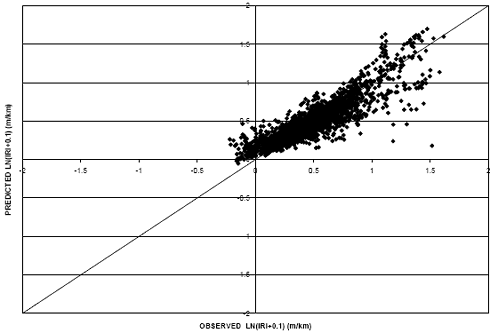1 m/km = 5.28 ft/mi

Figure 15. Scatter plot. Rigid IRI model (shifted).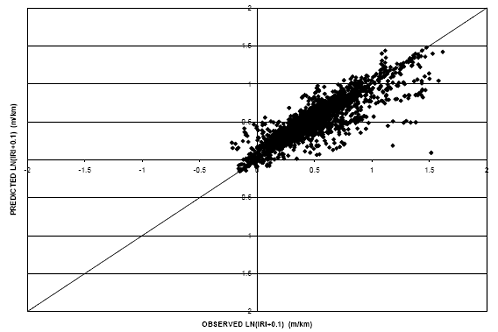1 m/km = 5.28 ft/mi

Different relationships between the performance measure, IRI, and pavement age were evaluated to ensure the model with the best prediction capability was selected for use in the environmental comparisons. Two models using different IRI-pavement age relationships were generated. The first model used a linear relationship between IRI and pavement age. Figure 16 provides a scatter plot of the predicted IRI values (shifted) versus the observed IRI values in the flexible dataset. An additional model was generated using an exponential relationship between IRI and pavement age. It is shown in figure 17 for the flexible dataset.

Using a linear relationship between IRI and age results in a model that is less biased than the exponential relationship. This is evident when comparing figures 16 and 17. In figure 16 the cluster of data points is more centered on the equality line as compared with figure 17 where the majority of the cluster falls below the line. This indicates a model that is generally predicting values less than the observed values. Further, the RMSE value for the linear model was considerably lower (0.17) than the exponential model (0.33). It is also interesting to note that the exponential model does not predict an increase in the IRI over time (as can be seen in figure 18). For these reasons, the linear relationship model was selected for consideration in the environmental comparisons. A scatter plot of predicted (shifted) versus observed values using the linear age relationship for the rigid dataset appears in figure 19.

Figure 16. Scatter plot. Flexible IRI model with linear IRI-age relationship.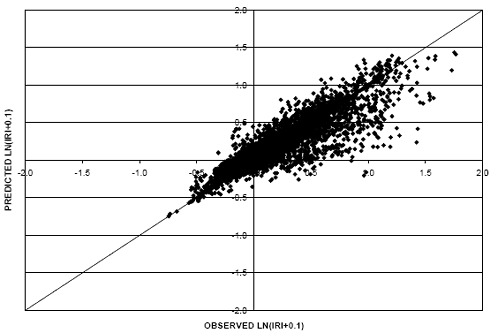Figure 17. Scatter plot. Flexible IRI model with IRI-exponential age relationship.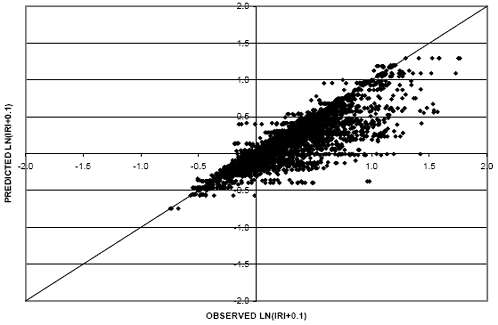Figure 18. Scatter plot. Actual and predicted IRI values for test section 011001 using IRI-exponential age relationship model.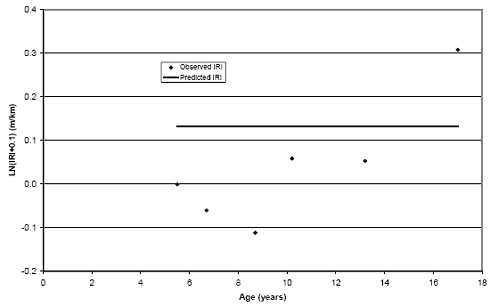1 m/km = 5.28 ft/mi

Figure 19. Scatter Plot. Rigid IRI model with linear IRI-age relationship.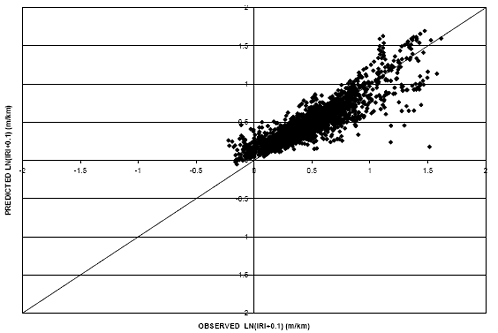1 m/km = 5.28 ft/mi

Equations representing the selected IRI models for both flexible and rigid pavements can be found in appendix B. The R-squared value for the flexible pavement IRI model (shifted) using the linear relationship between age and IRI is approximately 0.78 (P-value < 0.0001). The rigid pavement IRI model exhibited an R-squared of 0.78 (P-value < 0.0001).

### RUTTING PREDICTION MODELS FOR FLEXIBLE PAVEMENTS

Two models were considered in the prediction of rut depth in flexible pavements. The first model incorporated a linear relationship between rut depth and pavement age. A scatter plot of predicted versus observed rut depth values for this model is shown in figure 20. It resulted in a RMSE value of 0.59. The second model under consideration for use in predicting rut depth incorporated a natural logarithm relationship between rut depth and pavement age. The scatter plot of predicted versus observed values can be found in figure 21 corresponding to an RMSE value of 0.55.

Figure 20. Scatter plot. Rut depth model with linear rut-age relationship.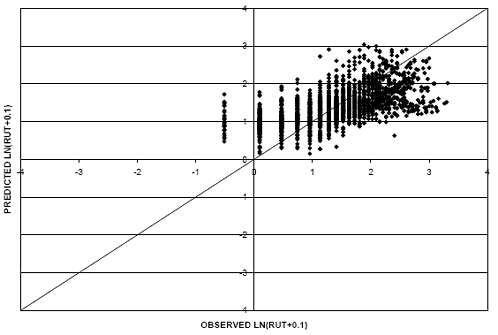Figure 21. Scatter plot. Rut depth model with rut-natural logarithm age relationship.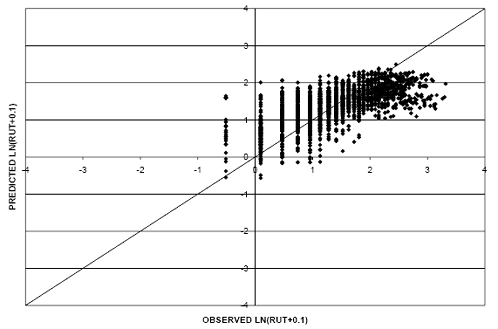The second model was selected for use in comparing rut depth performance of pavement in different environmental regions because of the improved fit of the dataset. Rutting mechanisms generally result in an increased rate of rutting in the early years of pavement life. As the pavement ages, this rate diminishes and rutting values level off (following a logarithmic relationship). Applying the logarithmic relationship in the model provides a better representation of the dataset, which is evident in the improved RMSE value. Details on this model can be found in appendix B. The adjusted R-squared value for this model was approximately 0.45 (P-value < 0.0001).

### SURFACE DISTRESS PREDICTION MODELS FOR BOTH FLEXIBLE AND RIGID PAVEMENTS

To develop the flexible dataset for distress measures, all three severity levels for each distress type were combined through the use of deduct curves developed for the South Dakota Department of Transportation(10) to obtain a deduct value for each distress. The study considered FC, BC, LWP, and TC. Because LWP often progresses to FC, the two distress types were combined into FWPC). LWP was converted from a linear unit to a unit of area to be consistent with FC. This was done by applying a standard width of 0.3 m (1 ft) to the recorded length of LWP. All severities of LWP were considered as low severity to compute deduct values that would be combined with the FC.

The format of distress data collected on rigid pavements does not match the required format used in the established deduct curves;(8) therefore, the severity levels were summed for each distress type. This total distress was then normalized based on the size of the test section in the same manner as the flexible sections. CB, LC, TC, and PUMP distress types were used in the rigid dataset.

Figure 22 provides a scatter plot of FWPC as a function of pavement age. As can be seen from the figure, there is a large amount of variability in the data, and numerous zeroes are recorded across the entire range of ages. For these reasons, regression models alone did not provide a good correlation with the measured values.

A small subset of the measured FWPC values was plotted (figure 23). Each series in the figure represents data from one test section. It appears that a substantial portion of the variability in the data can be attributed to the differences in age at which distress initiates. For example, distress initiation occurs just after construction at two of the sections, while another section does not initiate distress until age 17. There does appear to be a reasonable trend in the accumulation of distress with age after the initiation of distress.

Figure 22. Scatter plot. Measured FWPC deduct values.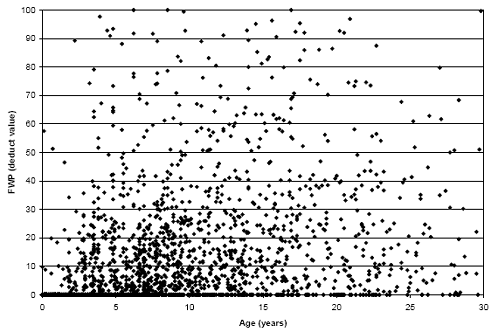Figure 23. Graph plot. Measured FWPC values (using a subset of test sections).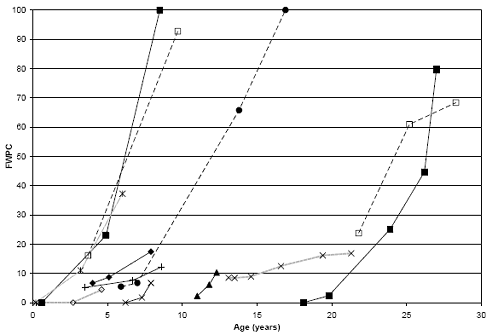Given the nature and form of the distress accumulation with age, two models were used concurrently to predict distress progression. The first model was used to predict the age at which distress initiation occurs, while the second estimated the accumulation of distress with age (after initiation).

The first model was developed using logistic analysis to predict age at which distress first appears. Logistical models predict the probability of an event occurring (e.g., distress initiation or nonzero distress value) given a set of variables including pavement age. Figure 24 shows an example of a logistic model. A cutoff probability must be established to predict an initiation age from the given model. As the cutoff probability increases, the accuracy of the model predicting events goes down, while the accuracy of predicting nonevents goes up; therefore, the selection of the cutoff probability depends on the nature of the data and the relative importance of events compared to nonevents. In the case of distress prediction, events and nonevents are of equal importance, so a cutoff value was selected that predicted each with equal accuracy. To determine the initiation age, all inputs for the logistic model are held constant (for a particular pavement section) except for pavement age, which is increased until the predicted probability is equivalent to the selected cutoff probability. This pavement age is defined as the initiation age. Table 19 is provided as an example illustrating the effect of the probability level on the accuracy of the model. The sensitivity denotes the percentage of events correctly identified, while the specificity reflects the nonevent accuracy. As the probability increases, the sensitivity decreases and the specificity increases. These two measures are approximately equal at the 0.7 probability level indicating events and nonevents, and are predicted with equal accuracy; therefore, 0.7 was established as the cutoff probability.

Figure 24. Graph plot. Example of logistical analysis to predict distress initiation.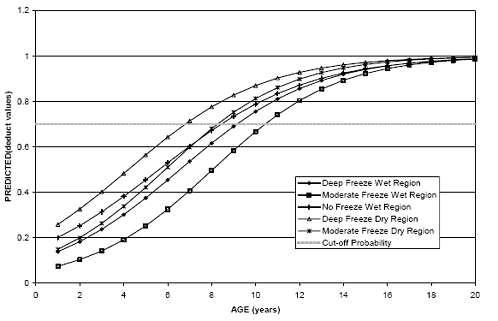Table 19. Example of probability level effect on logistic prediction.
Probability LevelCorrectIncorrectPercentages
EventNon-EventEventNon-EventCorrectSensitivitySpecificityFalse POSFalse NEG
0.113701606069.3100.00.230.70.0
0.2136560547572.199.69.928.67.7
0.313571794281377.799.129.524.06.8
0.413262723354480.896.844.820.213.9
0.5123236124613880.689.959.516.627.7
0.6112842917824278.882.370.713.636.1
0.795548012741572.669.779.111.746.4
0.87535179061764.255.085.210.754.4
0.95115565185954.037.391.69.160.7
1.006070137030.70.0100.0NA69.3

After the crack initiation age is predicted, linear regression models were used to predict the accumulation of distress with age (after initiation). The example in figure 24 indicates that the distress initiation ranges from approximately 7 years to more than10 years for the different environmental regions.

The pavement age variable in the dataset had to be adjusted to reflect age after distress initiation to develop the regression models used to predict surface distress. Two methodologies were used to adjust pavement age depending on the timing of distress initiation relative to the pavement monitoring period.

Some of the test sections were monitored both before and after crack initiation. For these cases, crack initiation was directly determined as the maximum pavement age where a zero distress value was observed. This crack initiation age was then used to adjust the remaining pavement ages to ages after distress initiation. An example of one such test section is presented in figure 25. The crack initiation age was determined to be 2.4 years. The remaining ages were then adjusted by subtracting 2.4 years from the pavement age to obtain age after initiation.

Figure 25. Graph plot. Observed FWPC deduct values for test section 100102.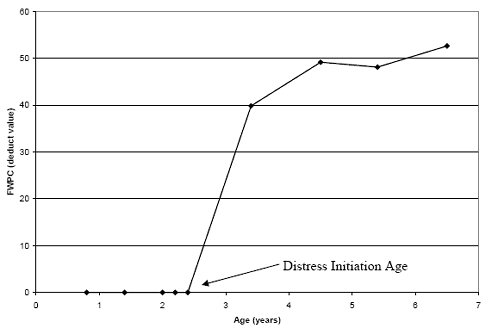For test sections that were not monitored before the distress initiation, linear regression was performed on each test section and used to determine the age at which the distress was initiated. An example of this is shown graphically in figure 26. The initiation age estimated from the regression equation was subtracted from subsequent pavement ages to get the age after initiation. The regression models were developed using only nonzero distress values (i.e., values recorded after initiation) and replacing age with these calculated adjusted age values.

Figure 26. Graph plot. Observed FWPC deduct values for test section 050121(with regression line).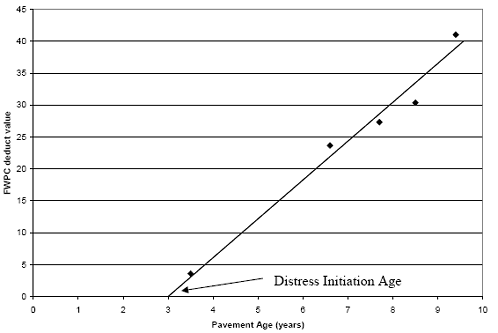Similar to other performance measures, multiple regression models were developed for each distress type using different distress-age relationships. These models were evaluated to select the model that predicts the observed values with the best accuracy. The first model incorporated a linear relationship between distress and pavement age while the second model was developed using a natural logarithm relationship.

Figure 27 provides a scatter plot of observed versus predicted values for the FWPC model using the logistic analysis coupled with the linear distress/pavement age regression model. Figure 28 shows a scatter plot for values generated from the regression model with the natural logarithmic relationship.

The vertical lines on the left side and the horizontal lines on the bottom of both figures indicate the error within the logistic analysis. The data points on the vertical lines (above the line of equality) are a result of the logistic analysis predicting distress initiation earlier than it was actually observed. Conversely, instances where the logistic analysis predicted crack initiation later than observed appear as data points on the horizontal line to the right of the line of equality. Although it looks as if only one of these data points falls on the line of equality, in actuality approximately 1,800 of the 2,400 points predicted using the logistic analysis were classified correctly as events or nonevents. Because the probability level of 0.7 resulted in equal sensitivity and specificity for the logistic model, it was selected as the cutoff probability. The vertical lines on the right side and the horizontal line on the top of both figures are the results of establishing a maximum allowable deduct value of 100.

Figure 27. Scatter plot. FWPC model for flexible pavements with linear FWPC-age relationship.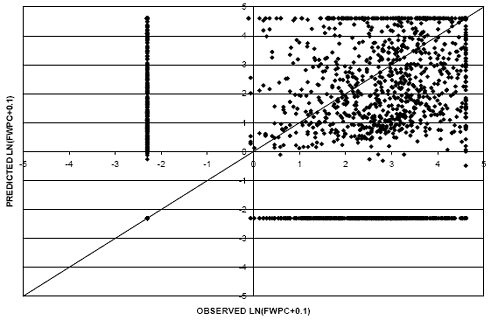Figure 28. Scatter plot. FWPC model for flexible pavements with FWPC-natural logarithm age relationship.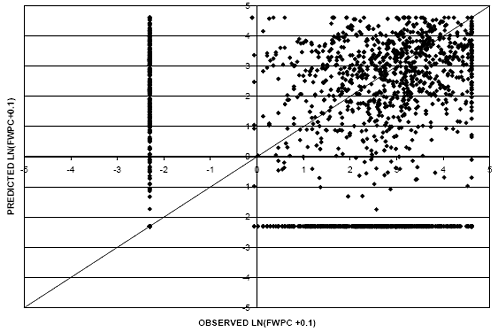In comparing the two models, the cluster of data points in figure 28 are more densely clustered around the line of equality. The linear regression model exhibits a larger RMSE value (1.85) as compared with the logarithmic regression model (1.41). In addition, the linear relationship model overpredicts a larger percentage of data points compared with the logarithmic relationship (evident in the horizontal line on the top of figure 27). For these reasons, the natural logarithmic relationship was selected for use in the environmental comparisons.

Appendix B provides details on the logistic and regression models used to predict FWPC. The R-squared value for the regression model was 0.63 (P-value < 0.0001). In addition, a regression model was developed for FWPC accumulation in terms of percentage of wheelpath area, and it was used in the description of application to mechanistic design (chapter 10). The R-squared value for this regression model was 0.49 (P-value < 0.0001).

Figures 29 and 30 provide scatter plots for TC models using linear and logarithmic relationships, respectively. To evaluate the logistic analysis, approximately 1,900 out of 2,400 records were accurately categorized as events or nonevents by the logistic model. This was achieved with a cutoff probability of 0.6. Similar to the FWPC models, the logarithmic relationship model exhibits an improved RMSE value of 1.21 as compared to a value of 1.69 for the linear model; therefore, the natural logarithmic relationship model was selected for use in the environmental comparisons.

Appendix B contains information on the TC logistic and regression models. The adjusted R-squared value for the TC regression model is approximately 0.71 (P-value < 0.0001).

Figure 29. Scatter plot. TC model for flexible pavements with linear TC-age relationship.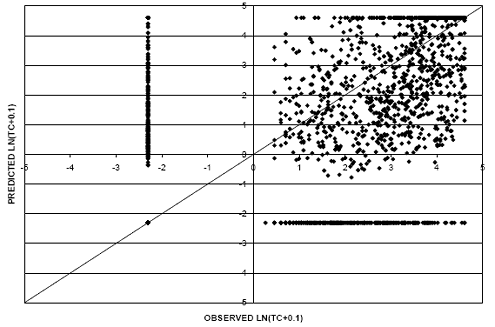Figure 30. Scatter plot. TC model for flexible pavements with TC-natural logarithm age relationship.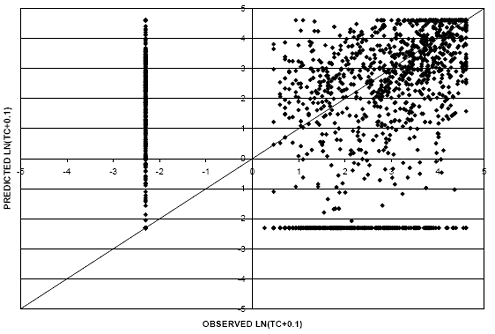Additional regression models were developed for block cracking. The regression models include a negative coefficient for pavement age. This indicates a reduction in pavement distress with increases in pavement age (under certain environmental conditions). The model’s poor correlation and negative distress age relationship can be attributed to the relatively small percentage of records in the dataset with nonzero BC deduct values (approximately 90 of the 2,400). In addition, it is probable that rather variability contributed to the regression results. Due to the subjective nature of data collection, an area of distress could be rated as block cracking during one data collection visit and subsequently rated as a series of longitudinal and transverse cracking on the next survey. This would result in a reduction in block cracking quantities with age. Therefore, given these issues as well as the results of the regression model, block cracking models were not evaluated as part of the performance comparisons.

CB was modeled using data from the rigid pavements selected for use in this study. Scatter plots for the linear and logarithmic CB-age relationship models appear in figures 31 and 32, respectively.

Figure 31. Scatter plot. CB model for rigid pavements with linear CB-age relationship.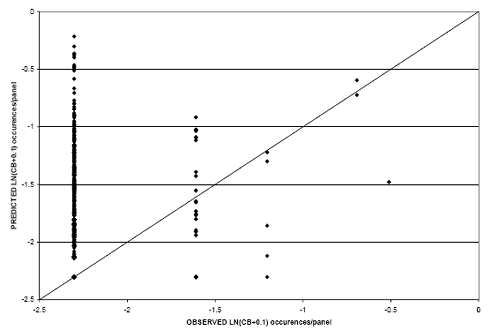Figure 32. Scatter plot. CB model for rigid pavements with CB-natural logarithm age relationship.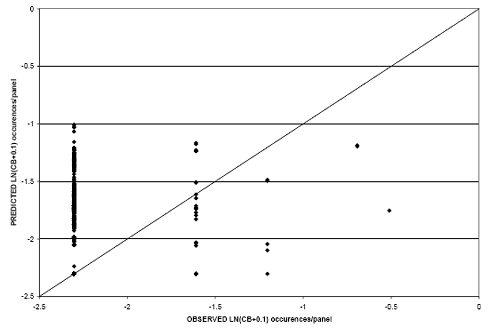As can be seen from the figures, both models provide poor correlation between predicted and observed values. This is caused, in part, by the limited number of nonzero observations in the dataset (approximately 3 percent of the records) in which the regression analysis was developed. The quantity of data points used in the regression was not large enough to account for the contribution of each explanatory variable. As such, the models developed from CB data were not used to make performance comparisons.

Additional analysis was performed on the rigid dataset to predict longitudinal cracking (LC). Consistent with the other distress performance measures, two models with different relationships between LC and pavement age were developed. Scatter plot results from the linear relationship model can be found in figure 33, while figure 34 provides similar results for the logarithmic model. The RMSE values for the linear and logarithmic relationship models were determined to be 1.49 and 1.42, respectively.

Because the model developed using the logarithmic relationship between LC and pavement age provides less variability compared with the linear relationship, it was selected for use in the performance comparison analysis. The logistic model correctly classified approximately 990 of the 1,350 records in the dataset. A cutoff probability of 0.55 was selected to determine initiation age. The regression portion of the model exhibits an R-squared value of 0.38 (P-value < 0.0001). Appendix B provides equations detailing the logistic and regression models for LC performance on rigid pavements.

Figure 33. Scatter plot. LC model for rigid pavements with linear LC-age relationship.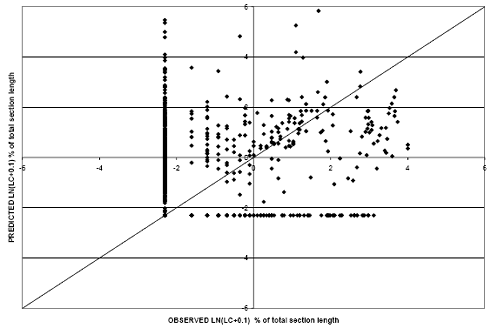Figure 34. Scatter plot. LC model for rigid pavements with LC-natural logarithm-age relationship.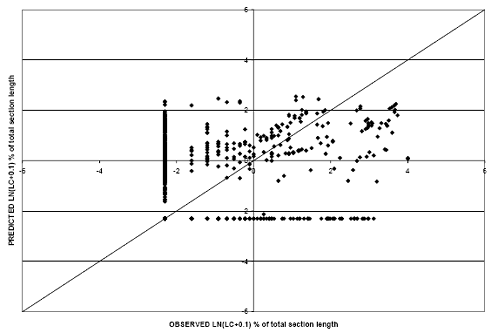Similar analysis was performed for TC of rigid pavements. Scatter plots for each of the models is provided in figures 35 and 36. As can be seen from the graphs, the logarithmic relationship model (RMSE equals 1.17) results in a better correlation between observed and predicted values as compared with the linear model (RMSE equals 1.24). The adjusted R-squared for the regression model is 0.53 (P-value < 0.0001) and the logistic analysis correctly categorized 920 of the 1,350 records in the dataset (at the selected cutoff probability of 0.6).

Figure 35. Scatter plot. TC model for rigid pavements with linear TC-age relationship.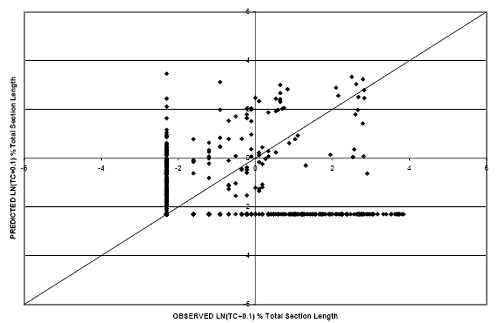Figure 36. Scatter plot. TC model for rigid pavements with TC-natural logarithm age relationship.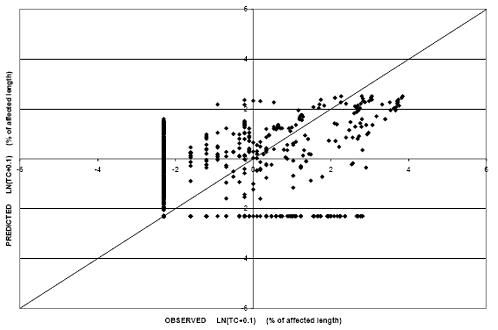Last, PUMP was modeled for the rigid pavement dataset. It should be noted that there was limited accumulation of pumping in the dataset. Approximately 110 records (8 per-cent) of the dataset exhibited nonzero PUMP values. The number of nonzero records is relatively small compared with the number of explanatory variables considered in the regression developed. Figure 37 provides the prediction capabilities of the model developed with a linear relationship between PUMP and pavement age. Figure 38 shows the results from the logarithmic model. Predicted values from the models do not correlate well with observed values, and therefore, they were not used to make performance comparisons.

Figure 37. Scatter plot. PUMP model for rigid pavements with linear PUMP-age relationship.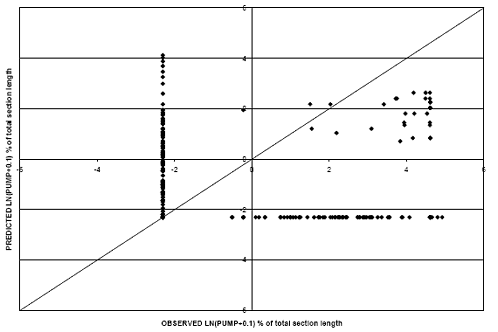Figure 38. Scatter plot. PUMP model for rigid pavements with PUMP-natural logarithm age relations.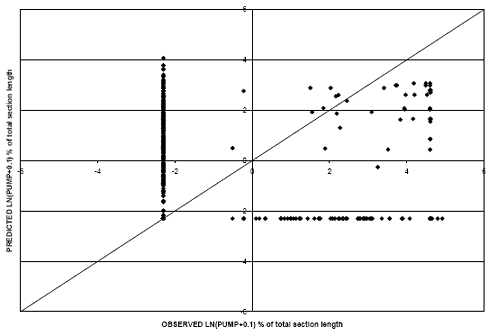### TRANSVERSE JOINT FAULTING PREDICTION MODELS FOR RIGID PAVEMENTS

Faulting provides an indication of the joint integrity (load transfer efficiency) as well as the condition of the underlying unbound layers. In addition, pavement roughness is directly affected by the magnitude of faulting, and performance models were developed for transverse joint faulting of rigid pavements. The average faulting value for all joints on a test section was established as the performance measure.

Two models were developed to predict the accumulation of faulting (FLT) with pavement age. The first model incorporated a linear relationship between the performance measure and pavement age; the second regression was developed with a logarithmic relationship. Figure 39 provides the scatter plot for the linear relationship model. Figure 40 represents the observed versus predicted values for the logarithmic relationship model.

The RMSE for the linear relationship model is approximately 0.37, which is an improvement over the logarithmic model (RMSE equal to 0.39). In addition, the logarithmic model predicted a decrease in accumulated faulting with an increase in pavement age in some cases. This is not logical because faulting should not improve as the pavement structure ages and is exposed to traffic loading. In consideration of this fact, as well as the improved goodness of fit, the linear relationship model was selected for use in the study.

Details regarding the model, which resulted in an adjusted R-squared value of 0.47 (P-value < 0.0001), appear in appendix B.

Figure 39. Scatter plot. FLT model for rigid pavements with linear FLT-age relationship.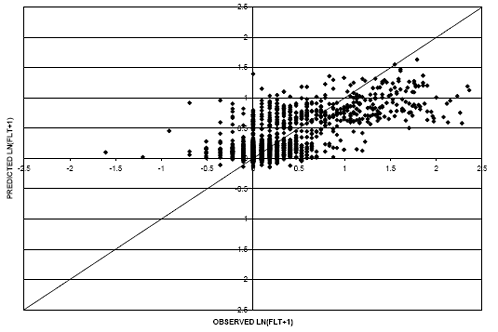Figure 40. Scatter plot. FLT model for rigid pavements with FLT-natural logarithm age relationship.